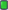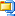Thread: Tools for Repacking View Single Post
#71MasqueradeRegistered User Join Date: Jan 2020 Location: Monte d'Or Posts: 748 Thanks: 168 Thanked 796 Times in 394 PostsForza Horizon 3 Filename Cipher
Code:
```l = a
` = b
^ = c
6 = d
q = e
v = f
{ = g
@ = h
\$ = i
7 = j
s = k
b = l
g = m
8 = n
h = o
u = p
f = q
4 = r
~ = s
1 = t
= = u
' = v
m = w
] = x
! = y
, = z
y = _
_ = -
[ = 0
0 = 1
w = 2
k = 3
( = 4
2 = 5
j = 6
} = 7
; = 8
+ = 9```
Forza Horizon 3 Filename Decipher Tool
• A little tool I wrote in C# to make reading the folders and file names from FH3 significantly easier• Usage:
Code:
`FH3FilenameDecipherTool.exe <input string>`
• Give encrypted input string to receive an output in plain English.
Attached FilesFH3FilenameDecipherTool.7z (2.4 KB, 5 views)

Last edited by Masquerade; 09-03-2022 at 11:24.